There are 366 different Starters of The Day, many to choose from. You will find below some starters on the topic of Place Value. A lesson starter does not have to be on the same topic as the main part of the lesson or the topic of the previous lesson. It is often very useful to revise or explore other concepts by using a starter based on a totally different area of Mathematics.

Main Page

Place Value Starters: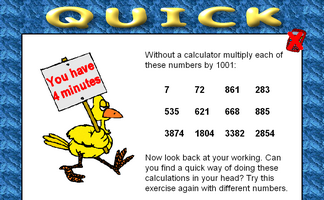Develop a quick way of mentally multiplying any number by 1001.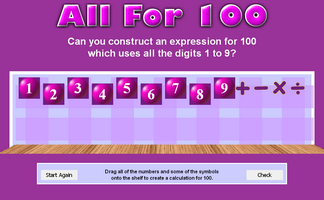Can you write an expression for 100 which uses all the digits 1 to 9?Estimate or calculate then put the large numbers in order of size.Use only the 1, 5 and 0 keys on a calculator to make given totals.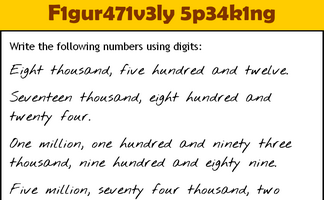Write the numbers written as words using digits.A little lateral thinking will help you solve this number puzzle.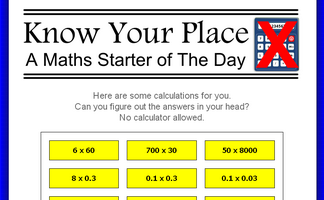Without a calculator perform some calculations requiring a knowledge of place value.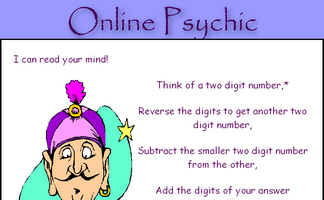Work out why subtracting a two digit number from its reverse gives a multiple of nine.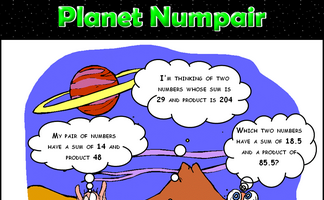Arrange the digits one to nine to make a correct addition calculation.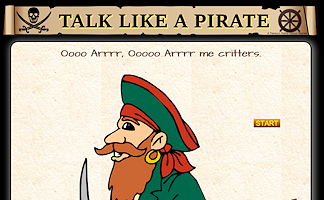Write out in words some numbers writen as digits (optional pirate theme)How many ways can you write an expression for 100 which only uses the same digit repeated and any operations?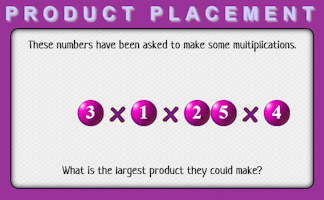Arrange the numbers to produce the largest product.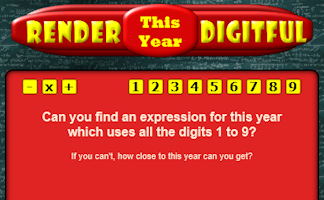Find a calculation for the current year which uses all of the digits 1 to 9.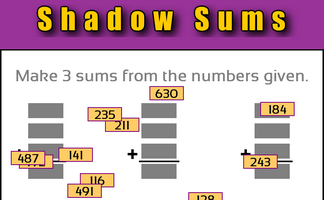Make sums from the three digit numbers given.Can you work out what each of the strange symbols represents in these calculations?Complete some imaginary cheques, the amount needs to be written in words.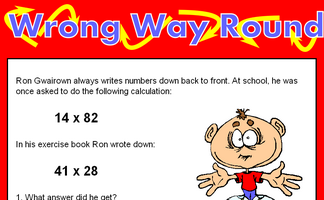Find calculations which written back to front give the same answer.

Advanced Place Value Starters

Search

The activity you are looking for may have been classified in a different way from the way you were expecting. You can search the whole of Transum Maths by using the box below.

Have today's Starter of the Day as your default homepage. Copy the URL below then select
Tools > Internet Options (Internet Explorer) then paste the URL into the homepage field.

Set as your homepage (if you are using Internet Explorer)

Do you have any comments? It is always useful to receive feedback and helps make this free resource even more useful for those learning Mathematics anywhere in the world. Click here to enter your comments.For All: